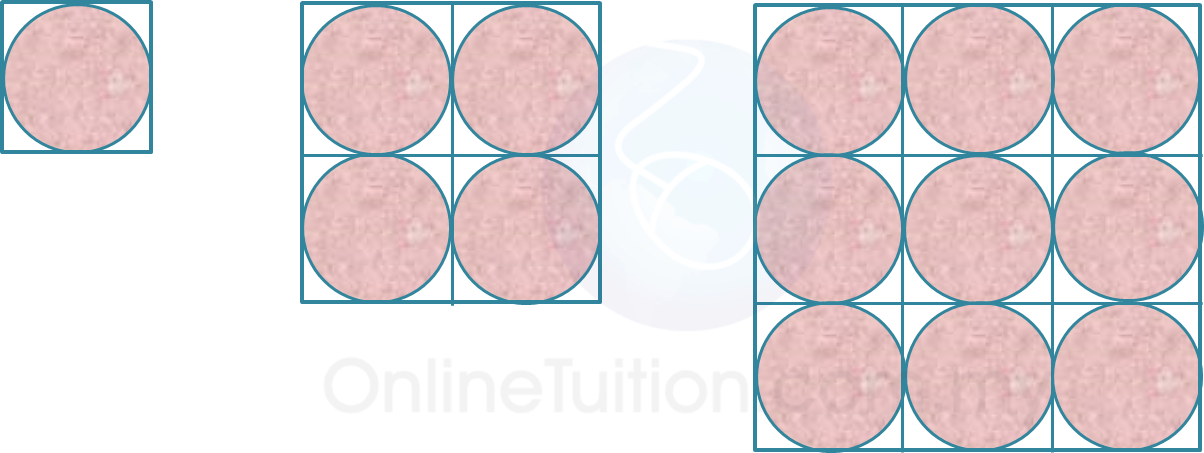# SPM Mathematics 2016, Paper 2 (Questions 7 & 8)

Question 7 (5 marks):
(a) State whether the following statements are true statement or false statement.

(b) Diagram 7 shows the first three patterns of a sequence of patterns.Diagram 7

It is given that the diameter of each circle is 20 cm.
(i) Make a general conclusion by induction for the area of the unshaded region.

(ii)
Hence, calculate the area of the unshaded region for the 5th pattern.

Solution:
(a)(i) True

(a)(ii) False

(b)(i)
= (20 × 20) – π(10)2
= 400 – 100π
= 100 (4 – π)

= 4 × 100 (4 – π)
= 400 (4 – π)

= 9 × 100 (4 – π)
= 900 (4 – π)
100 (4 – π), 400 (4 – π), 900 (4 – π), …
102 (4 – π), 202 (4 – π), 302 (4 – π), …

General conclusion = n2 (4 – π)
n = 10, 20, 30, …

(b)(ii)

= 502 (4 – π)
= 2500 (4 – π)

Question 8 (5 marks):
Table 8 shows the information of books purchased by Maslinda.Table 8

Maslinda purchased x history books and y Science books. The total number of books purchased is 5. The total price of the books purchased is RM17.

(a)
Write two linear equations in terms of x and y to represent the above information.

(b)
Hence, by using matrix method, calculate the value of x and of y.

Solution:
(a)
x + y = 5
4x + 3y = 17

(b)